2022: SklogWiki celebrates 15 years on-line

# Canonical ensemble

Variables:

• Number of Particles,$N$
• Volume,$V$
• Temperature,$T$

## Partition Function

The partition function,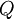$Q$, for a system of$N$ identical particles each of mass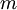$m$ is given by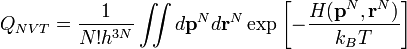$Q_{NVT}=\frac{1}{N!h^{3N}}\iint d{\mathbf p}^N d{\mathbf r}^N \exp \left[ - \frac{H({\mathbf p}^N,{\mathbf r}^N)}{k_B T}\right]$

where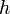$h$ is Planck's constant,$T$ is the temperature,$k_B$ is the Boltzmann constant and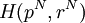$H(p^N, r^N)$ is the Hamiltonian corresponding to the total energy of the system. For a classical one-component system in a three-dimensional space,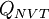$Q_{NVT}$, is given by: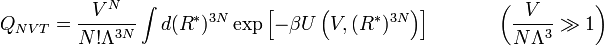$Q_{NVT} = \frac{V^N}{N! \Lambda^{3N} } \int d (R^*)^{3N} \exp \left[ - \beta U \left( V, (R^*)^{3N} \right) \right] ~~~~~~~~~~ \left( \frac{V}{N\Lambda^3} \gg 1 \right)$

where:

•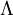$\Lambda$ is the de Broglie thermal wavelength (depends on the temperature)
•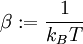$\beta := \frac{1}{k_B T}$, with$k_B$ being the Boltzmann constant, and T the temperature.
•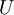$U$ is the potential energy, which depends on the coordinates of the particles (and on the interaction model)
•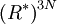$\left( R^*\right)^{3N}$ represent the 3N position coordinates of the particles (reduced with the system size): i.e.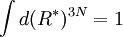$\int d (R^*)^{3N} = 1$